# RD Sharma Solutions for Class 6 Maths Chapter 5: Negative Numbers and Integers Exercise 5.1

## RD Sharma Solutions for Class 6 Maths Exercise 5.1 PDF

RD Sharma Solutions are useful for the students to score well in the exam for Class 6. The primary aim is to provide the students with chapter wise solutions which provide better knowledge about the concepts. The subject matter experts create the solutions based on the latest CBSE syllabus for Class 6. The solutions which are available in PDF format can be downloaded by the students. It helps students to understand the methods used in solving tricky problems and master the subject. RD Sharma Solutions for Class 6 Maths Chapter 5 Negative Numbers and Integers Exercise 5.1 are provided here.

## RD Sharma Solutions for Class 6 Maths Chapter 5: Negative Numbers and Integers Exercise 5.1 Download PDF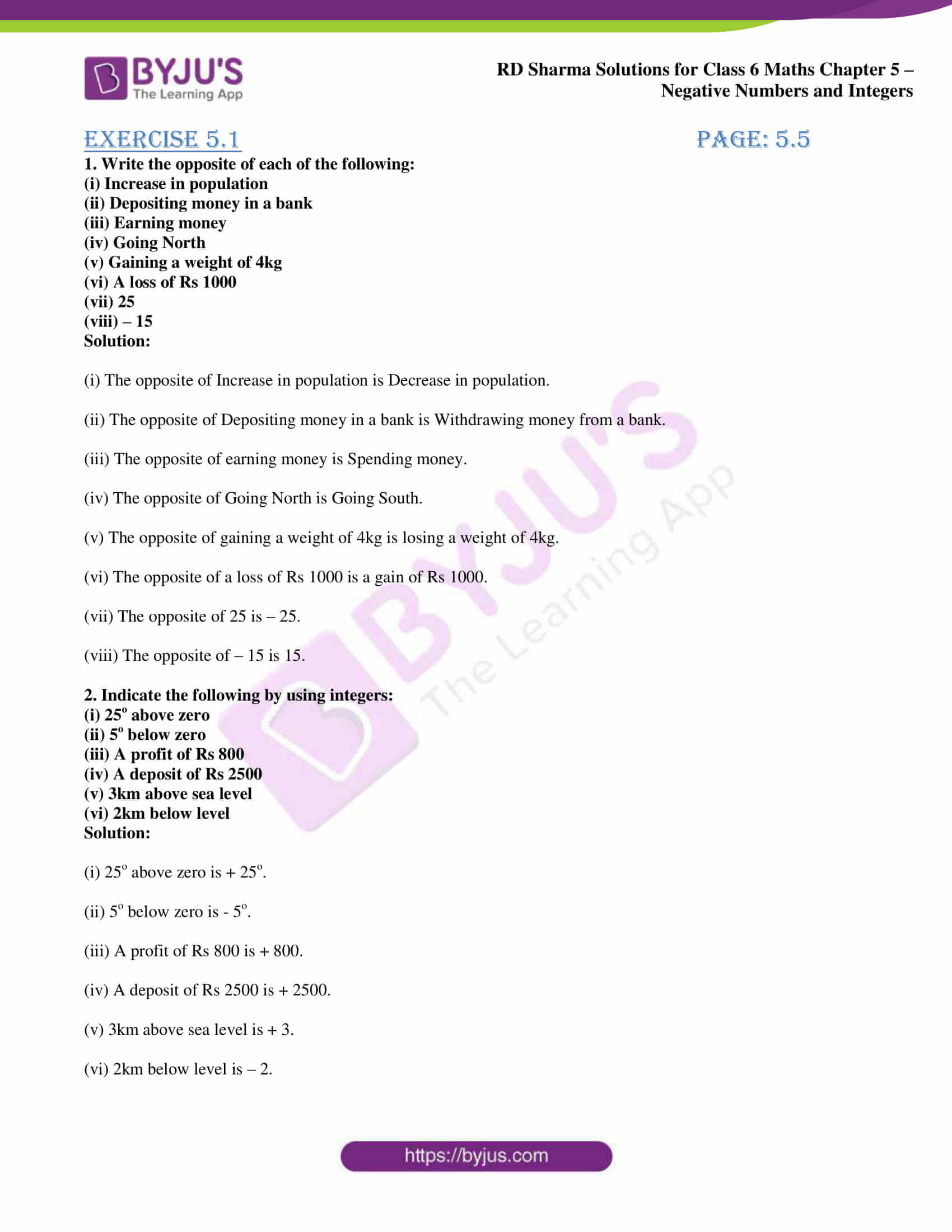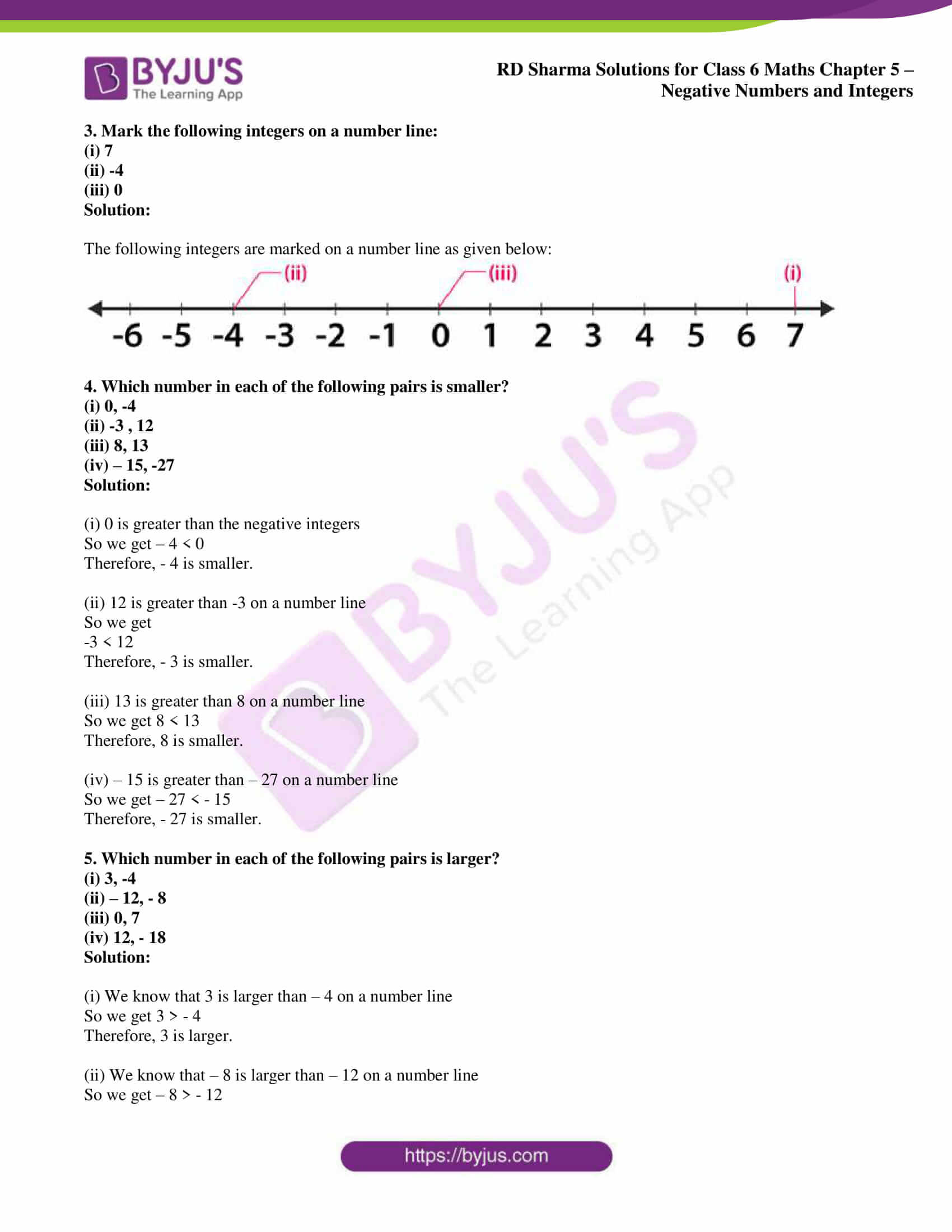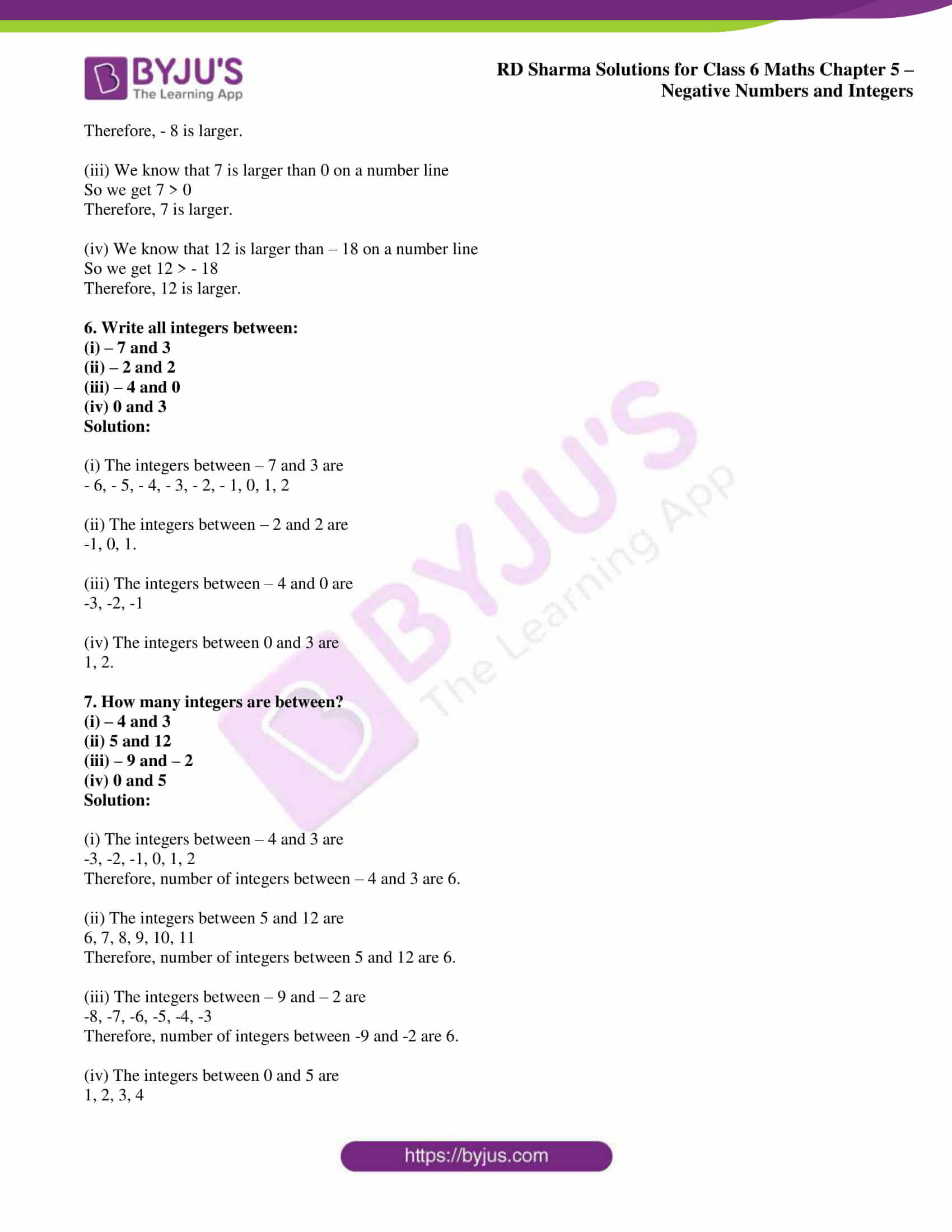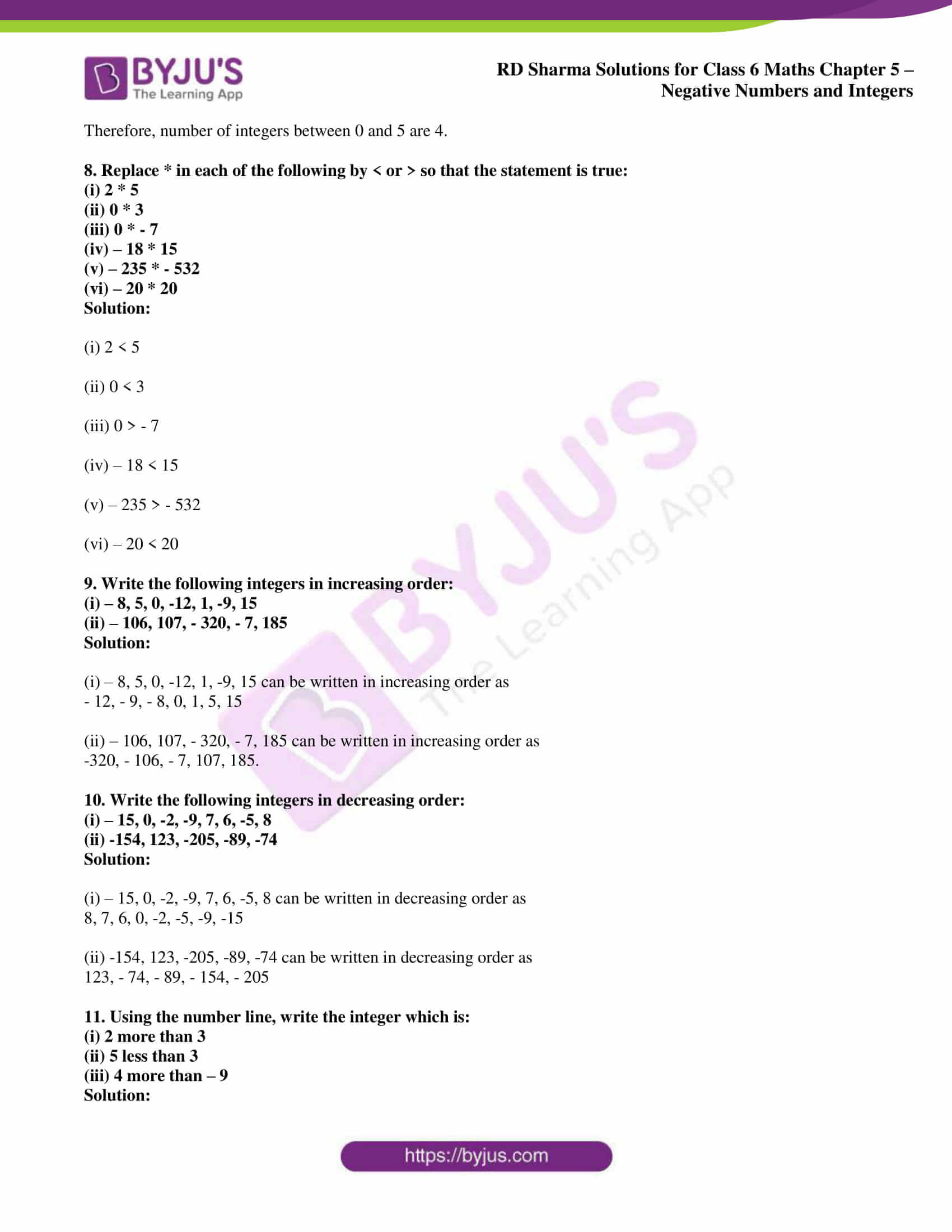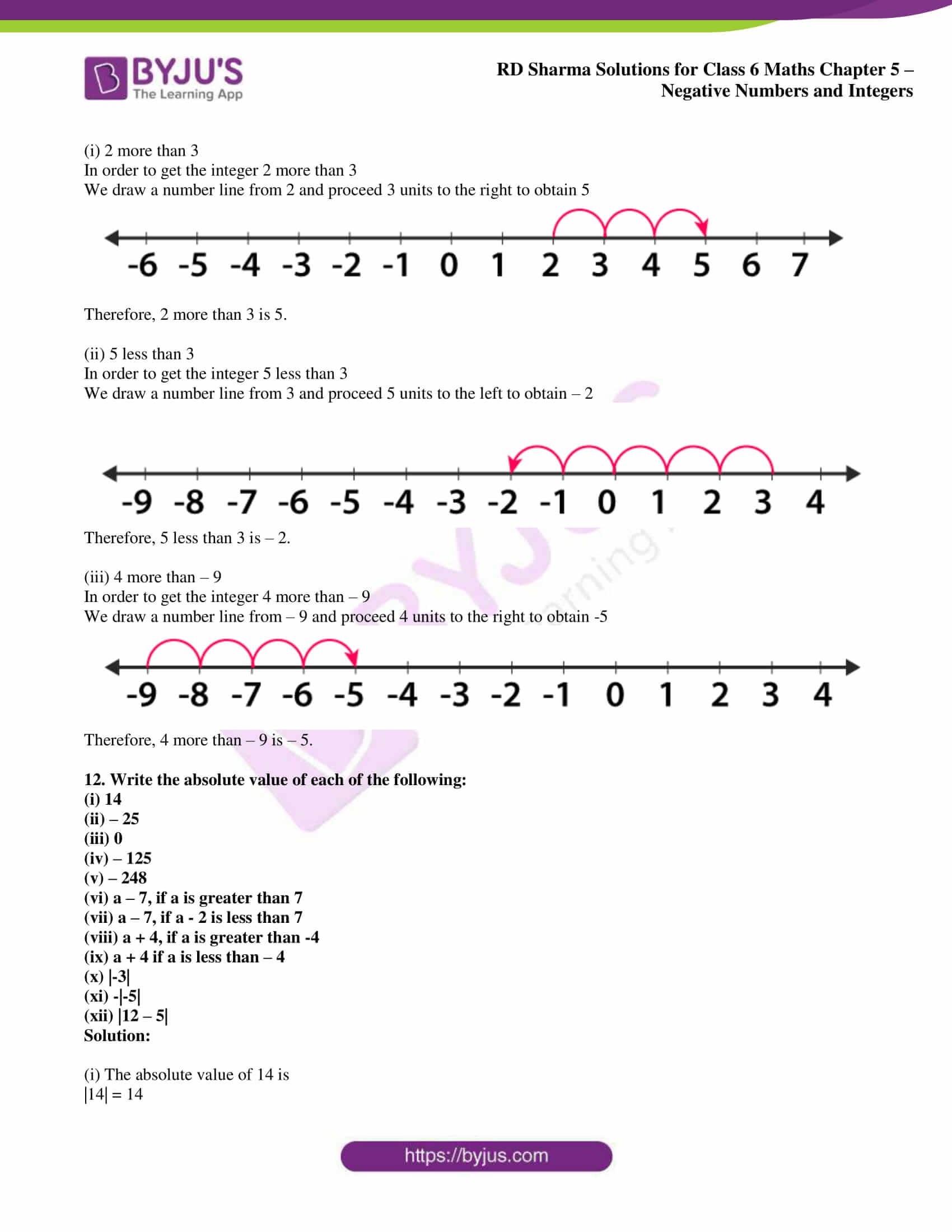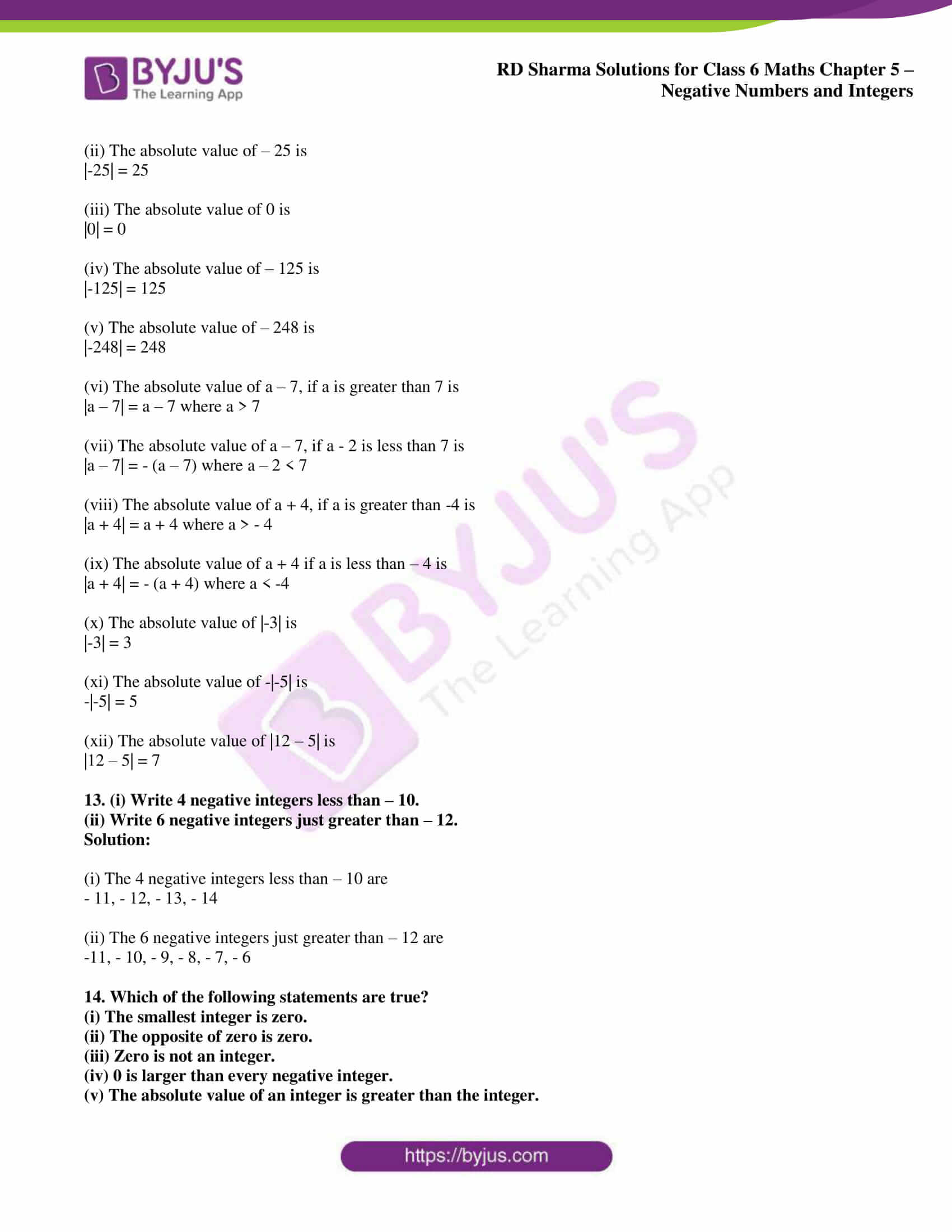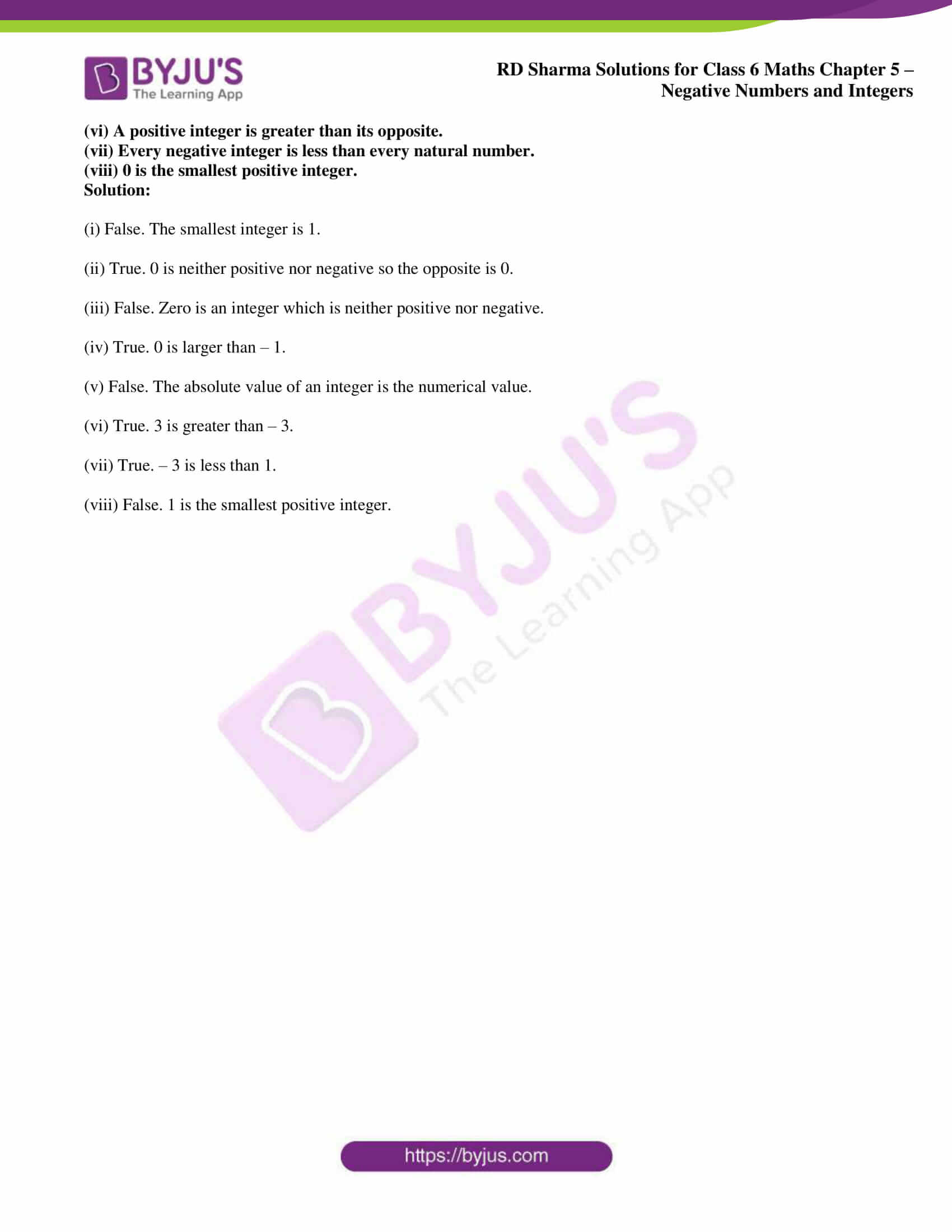## Access answers to Maths RD Sharma Solutions for Class 6 Chapter 5: Negative Numbers and Integers Exercise 5.1

1. Write the opposite of each of the following:

(i) Increase in population

(ii) Depositing money in a bank

(iii) Earning money

(iv) Going North

(v) Gaining a weight of 4kg

(vi) A loss of Rs 1000

(vii) 25

(viii) – 15

Solution:

(i) The opposite of Increase in population is Decrease in population.

(ii) The opposite of Depositing money in a bank is Withdrawing money from a bank.

(iii) The opposite of earning money is Spending money.

(iv) The opposite of Going North is Going South.

(v) The opposite of gaining a weight of 4kg is losing a weight of 4kg.

(vi) The opposite of a loss of Rs 1000 is a gain of Rs 1000.

(vii) The opposite of 25 is – 25.

(viii) The opposite of – 15 is 15.

2. Indicate the following by using integers:

(i) 25o above zero

(ii) 5o below zero

(iii) A profit of Rs 800

(iv) A deposit of Rs 2500

(v) 3km above sea level

(vi) 2km below level

Solution:

(i) 25o above zero is + 25o.

(ii) 5o below zero is – 5o.

(iii) A profit of Rs 800 is + 800.

(iv) A deposit of Rs 2500 is + 2500.

(v) 3km above sea level is + 3.

(vi) 2km below level is – 2.

3. Mark the following integers on a number line:

(i) 7

(ii) -4

(iii) 0

Solution:

The following integers are marked on a number line as given below: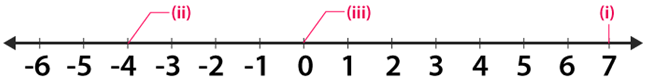4. Which number in each of the following pairs is smaller?

(i) 0, -4

(ii) -3 , 12

(iii) 8, 13

(iv) – 15, -27

Solution:

(i) 0 is greater than the negative integers

So we get – 4 < 0

Therefore, – 4 is smaller.

(ii) 12 is greater than -3 on a number line

So we get

-3 < 12

Therefore, – 3 is smaller.

(iii) 13 is greater than 8 on a number line

So we get 8 < 13

Therefore, 8 is smaller.

(iv) – 15 is greater than – 27 on a number line

So we get – 27 < – 15

Therefore, – 27 is smaller.

5. Which number in each of the following pairs is larger?

(i) 3, -4

(ii) – 12, – 8

(iii) 0, 7

(iv) 12, – 18

Solution:

(i) We know that 3 is larger than – 4 on a number line

So we get 3 > – 4

Therefore, 3 is larger.

(ii) We know that – 8 is larger than – 12 on a number line

So we get – 8 > – 12

Therefore, – 8 is larger.

(iii) We know that 7 is larger than 0 on a number line

So we get 7 > 0

Therefore, 7 is larger.

(iv) We know that 12 is larger than – 18 on a number line

So we get 12 > – 18

Therefore, 12 is larger.

6. Write all integers between:

(i) – 7 and 3

(ii) – 2 and 2

(iii) – 4 and 0

(iv) 0 and 3

Solution:

(i) The integers between – 7 and 3 are

– 6, – 5, – 4, – 3, – 2, – 1, 0, 1, 2

(ii) The integers between – 2 and 2 are

-1, 0, 1.

(iii) The integers between – 4 and 0 are

-3, -2, -1

(iv) The integers between 0 and 3 are

1, 2.

7. How many integers are between?

(i) – 4 and 3

(ii) 5 and 12

(iii) – 9 and – 2

(iv) 0 and 5

Solution:

(i) The integers between – 4 and 3 are

-3, -2, -1, 0, 1, 2

Therefore, number of integers between – 4 and 3 are 6.

(ii) The integers between 5 and 12 are

6, 7, 8, 9, 10, 11

Therefore, number of integers between 5 and 12 are 6.

(iii) The integers between – 9 and – 2 are

-8, -7, -6, -5, -4, -3

Therefore, number of integers between -9 and -2 are 6.

(iv) The integers between 0 and 5 are

1, 2, 3, 4

Therefore, number of integers between 0 and 5 are 4.

8. Replace * in each of the following by < or > so that the statement is true:

(i) 2 * 5

(ii) 0 * 3

(iii) 0 * – 7

(iv) – 18 * 15

(v) – 235 * – 532

(vi) – 20 * 20

Solution:

(i) 2 < 5

(ii) 0 < 3

(iii) 0 > – 7

(iv) – 18 < 15

(v) – 235 > – 532

(vi) – 20 < 20

9. Write the following integers in increasing order:

(i) – 8, 5, 0, -12, 1, -9, 15

(ii) – 106, 107, – 320, – 7, 185

Solution:

(i) – 8, 5, 0, -12, 1, -9, 15 can be written in increasing order as

– 12, – 9, – 8, 0, 1, 5, 15

(ii) – 106, 107, – 320, – 7, 185 can be written in increasing order as

-320, – 106, – 7, 107, 185.

10. Write the following integers in decreasing order:

(i) – 15, 0, -2, -9, 7, 6, -5, 8

(ii) -154, 123, -205, -89, -74

Solution:

(i) – 15, 0, -2, -9, 7, 6, -5, 8 can be written in decreasing order as

8, 7, 6, 0, -2, -5, -9, -15

(ii) -154, 123, -205, -89, -74 can be written in decreasing order as

123, – 74, – 89, – 154, – 205

11. Using the number line, write the integer which is:

(i) 2 more than 3

(ii) 5 less than 3

(iii) 4 more than – 9

Solution:

(i) 2 more than 3

In order to get the integer 2 more than 3

We draw a number line from 2 and proceed 3 units to the right to obtain 5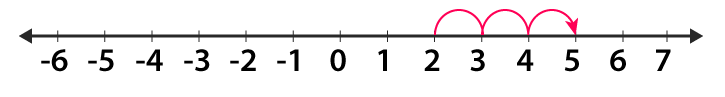Therefore, 2 more than 3 is 5.

(ii) 5 less than 3

In order to get the integer 5 less than 3

We draw a number line from 3 and proceed 5 units to the left to obtain – 2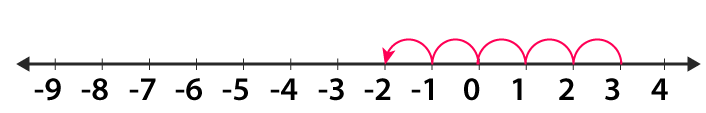Therefore, 5 less than 3 is – 2.

(iii) 4 more than – 9

In order to get the integer 4 more than – 9

We draw a number line from – 9 and proceed 4 units to the right to obtain -5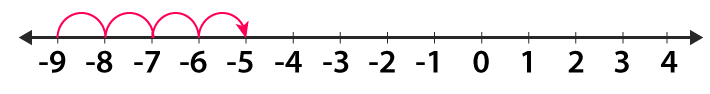Therefore, 4 more than – 9 is – 5.

12. Write the absolute value of each of the following:

(i) 14

(ii) – 25

(iii) 0

(iv) – 125

(v) – 248

(vi) a – 7, if a is greater than 7

(vii) a – 7, if a – 2 is less than 7

(viii) a + 4, if a is greater than -4

(ix) a + 4 if a is less than – 4

(x) |-3|

(xi) -|-5|

(xii) |12 – 5|

Solution:

(i) The absolute value of 14 is

|14| = 14

(ii) The absolute value of – 25 is

|-25| = 25

(iii) The absolute value of 0 is

|0| = 0

(iv) The absolute value of – 125 is

|-125| = 125

(v) The absolute value of – 248 is

|-248| = 248

(vi) The absolute value of a – 7, if a is greater than 7 is

|a – 7| = a – 7 where a > 7

(vii) The absolute value of a – 7, if a – 2 is less than 7 is

|a – 7| = – (a – 7) where a – 2 < 7

(viii) The absolute value of a + 4, if a is greater than -4 is

|a + 4| = a + 4 where a > – 4

(ix) The absolute value of a + 4 if a is less than – 4 is

|a + 4| = – (a + 4) where a < -4

(x) The absolute value of |-3| is

|-3| = 3

(xi) The absolute value of -|-5| is

-|-5| = 5

(xii) The absolute value of |12 – 5| is

|12 – 5| = 7

13. (i) Write 4 negative integers less than – 10.

(ii) Write 6 negative integers just greater than – 12.

Solution:

(i) The 4 negative integers less than – 10 are

– 11, – 12, – 13, – 14

(ii) The 6 negative integers just greater than – 12 are

-11, – 10, – 9, – 8, – 7, – 6

14. Which of the following statements are true?

(i) The smallest integer is zero.

(ii) The opposite of zero is zero.

(iii) Zero is not an integer.

(iv) 0 is larger than every negative integer.

(v) The absolute value of an integer is greater than the integer.

(vi) A positive integer is greater than its opposite.

(vii) Every negative integer is less than every natural number.

(viii) 0 is the smallest positive integer.

Solution:

(i) False. The smallest integer is 1.

(ii) True. 0 is neither positive nor negative so the opposite is 0.

(iii) False. Zero is an integer which is neither positive nor negative.

(iv) True. 0 is larger than – 1.

(v) False. The absolute value of an integer is the numerical value.

(vi) True. 3 is greater than – 3.

(vii) True. – 3 is less than 1.

(viii) False. 1 is the smallest positive integer.

### RD Sharma Solutions for Class 6 Maths Chapter 5 – Negative Numbers and Integers Exercise 5.1

RD Sharma Solutions Class 6 Maths Chapter 5 Negative Numbers and Integers Exercise 5.1 explains the concepts like positive and negative integers along with methods of representing integers on a number line.

### Key features of RD Sharma Solutions for Class 6 Maths Chapter 5: Negative Numbers and Integers Exercise 5.1

• The solutions created by the subject experts inculcate right attitude towards studies among students.
• The concepts are explained in a simple language to make the students clear with the basics.
• It helps students to increase their efficiency in solving exercise wise problems of RD Sharma textbook.
• The solutions are assembled with detailed explanations which help students in solving problems at their own pace.